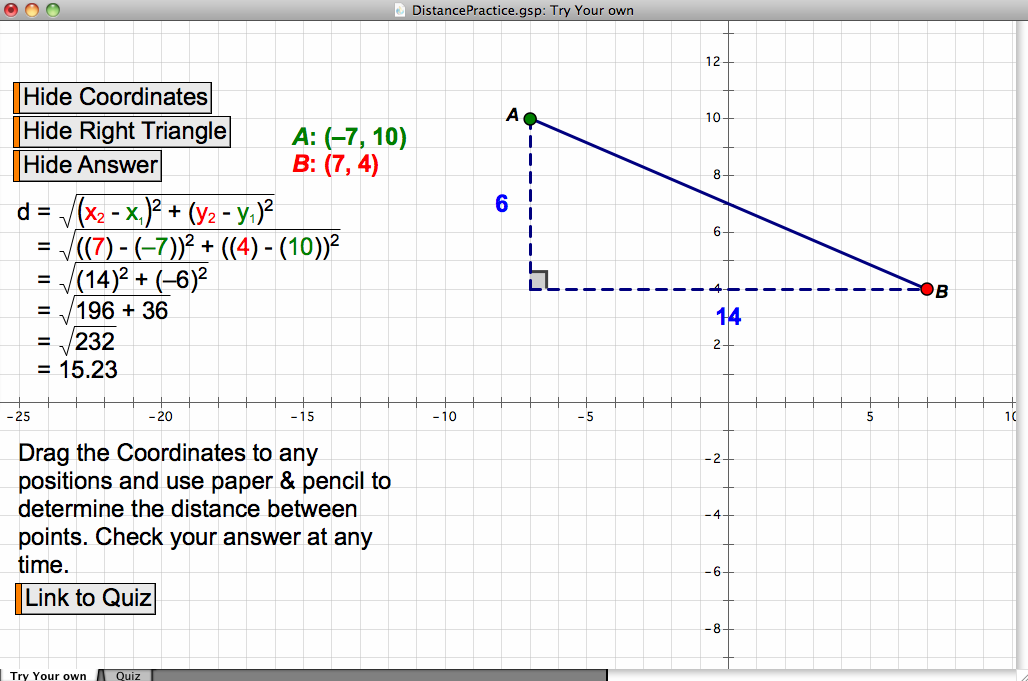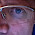## Wednesday, 29 October 2014

### Geometer's Sketchpad - Practice Distance Between PointsWhen using the Geometer's Sketchpad it is often better to "start from sketch, not from scratch". That is, give students a premade sketch rather having them build something from nothing (as many textbooks would have you do). In this activity students can download a GSP sketch that allows them to practice determining the distance between two points (this part could also be used to check answers) and then to be quizzed with randomly generated sets of points.

• MPM2D - develop the formula for the length of a line segment, and use this formula to solve problems (e.g., determine the lengths of the line segments joining the midpoints of the sides of a triangle, given the coordinates of the vertices of the triangle, and verify using dynamic geometry software);
• Note that this really works well on an iPad using the Sketchpad Explorer App (which is free)
• You can also use this on any web based computer (or Chromebook) with this Web sketch
Watch the video below to see how to use the sketch. The first page can be used for discovery or for checking problems and the second page can be used for quizzing students as it will generate an infinite number of random points to find the distance between.

Did you use this activity? Do you have a way to make it better? If so tell us in the comment section. Thanks

1.1.